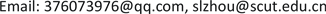﻿ Mathieu群与旗传递2-(v,k,λ)设计 Mathieu Groups and Flag-Transitive 2-(v,k,λ) Designs

Pure Mathematics
Vol.08 No.01(2018), Article ID:23503,8 pages
10.12677/PM.2018.81008

Mathieu Groups and Flag-Transitive $2-\left(v,k,\lambda \right)$ Designs

Jianan Chen, Shenglin Zhou

School of Mathematics, South China University of Technology, Guangzhou GuangdongReceived: Jan. 3rd, 2018; accepted: Jan. 17th, 2018; published: Jan. 24th, 2018ABSTRACT

Flag-transitivity is one of the important conditions that can be imposed on the automorphism group of a $2-\left(v,k,\lambda \right)$ design. The classification of flag-transitive 2-designs is an important problem in the algebraic combinatorial theory. Dembowski has proved that if $G\le Aut\left(\mathcal{D}\right)$ is flag-tran- sitive and $\left(v-1,k-1\right)\le 2$ , then $G$ is also point-primitive. According to this result, in this paper we completed the classification of this type of designs, with $Soc\left(G\right)$ was one of five Mathieu groups ${M}_{i}$ , where i =11, 12, 22, 23 or 24. We prove that there exists 62 2-designs satisfying the assumption.

Keywords:2-Design, Flag-Transitive, Socle, Mathieu GroupMathieu群与旗传递 $2-\left(v,k,\lambda \right)$ 设计Copyright © 2018 by authors and Hans Publishers Inc.1. 引言

1) $P$ 是有 $v$ 个点的有限集， $P$ 中的元素称为点；

2) $\mathcal{B}$$P$$b$$k$ -子集构成的集族， $\mathcal{B}$ 的元素称为区组或区；

3) $P$ 的任意给定的2-子集都恰好包含在 $\mathcal{B}$$\lambda$ 个区组之中。

1) $bk=vr$

2) $\lambda \left(v-1\right)=r\left(k-1\right)$

3) $b\ge v$

1) $G$ 是点–传递的，并且 ${G}_{\alpha }$$P\left(\alpha \right)$ 上传递,，其中 $P\left(\alpha \right)$ 表示所有过点 $\alpha$ 的区组；Table 1. 54 designs and parameters with ( v − 1 , k − 1 ) = 1

ContinuedTable 2. 8 designs and parameters with ( v − 1 , k − 1 ) = 2

2) $G$ 是区–传递的，并且 ${G}_{B}$ 在区组 $B$ 上传递。

1) $G$$\Omega$ 上是2-传递的；

2) $n=21$$G$${A}_{7}$${S}_{7}$ 作用在集合 $\left\{1,2,\cdots ,7\right\}$ 的无序二元组上；且非平凡的次级数为10。

1) ${r}^{2}>\lambda v\ge v$

2) $r||{G}_{\alpha }|$${G}_{\alpha }$$G$ 的点稳定子群， ${|{G}_{\alpha }|}^{3}>|G|$

3) $r|\lambda d$ ，其中 $d$ 表示 $G$ 的任一非平凡次轨道长度。特别地， $\frac{r}{\left(r,\lambda \right)}|d$

$\lambda \left(v-1\right)=r\left(k-1\right)$ ，即 $\lambda v-\lambda +r=rk$$v\le \lambda v<\lambda v-\lambda +r=rk\le {r}^{2}$

2) 由上式和引理2，我们有 $r||{G}_{\alpha }|$ ，所以 $r\le |{G}_{\alpha }|$$v<{r}^{2}\le {|{G}_{\alpha }|}^{2}$ ，又 $v=|G:{G}_{\alpha }|$ ，所以 $|G|<{|{G}_{\alpha }|}^{3}$

3) 设 $\alpha \in P$${G}_{\alpha }$ 的一个非平凡轨道为 $\Gamma$ ，长度为 $d$ 。令 $B$ 是过点 $\alpha$ 的一个区组， $m=|\Gamma \cap B|$ 。因为 $G$ 是旗传递的，所以 $m$$B$ 的选取无关。我们用两种不同的方法计数旗 $\left(\beta ,B\right)$$\beta \in \Gamma$$\alpha \in B$ ，即得 $rm=\lambda |\Gamma |$ 。所以， $r|\lambda d$

$\left(v-1,k-1\right)=1$ 时， $\left(v-1\right)|\frac{r}{\left(r,\lambda \right)}$ ，由引理4可知 $\frac{r}{\left(r,\lambda \right)}|d$ ，所以 $\left(v-1\right)|d$$\left(v-1\right)\le d$ 。又 $d$$G$ 的非平凡轨道长，显然 $\left(v-1\right)\ge d$ ，即 $d=v-1$ ，从而 ${G}_{\alpha }$$\Omega -\alpha$ 上传递，所以 $G$$\Omega$ 上2-传递。

$\left(v-1,k-1\right)=2$ 时， $\frac{v-1}{2}|\frac{r}{\left(r,\lambda \right)}|d$ ，所以 $d=\frac{v-1}{2}$ 或者 $d=v-1$ 。如果 $d=v-1$ ，那么 $G$$\Omega$ 上2-传递。如果 $d=\frac{v-1}{2}$ ，那么 $G$$\Omega$ 上3/2-传递秩3群。下证 $G$ 必定是本原的。当 $G$ 2-传递时，显然是本原的。当 $G$ 3/2-传递秩3群，假设 $G$ 是非本原的，则存在非本原块 $\Delta$ 使得 $\alpha \in \Delta$$1<|\Delta | 。但 $\Delta$${G}_{\alpha }$ 的一些轨道的并。设 ${G}_{\alpha }$ 的轨道为 $\left\{\alpha \right\}$${\Delta }_{1}$${\Delta }_{2}$ ，其中 $|{\Delta }_{1}|=|{\Delta }_{2}|=\frac{n-1}{2}$ ，则 $\Delta =\alpha \cup {\Delta }_{1}$$\Delta =\alpha \cup {\Delta }_{2}$ ，此时 $|\Delta |=1+\frac{n-1}{2}=\frac{n+1}{2}$ ，而 $\frac{n+1}{2}$ n，所以 $G$ 是本原的。

2. 定理1的证明

2.1. 可能的参数

$G={M}_{i}\left(i=11,12,22,23,24\right)$ ，为使得设计是非完全的，那么必有 $k\ge 5$ 。需要知道的是， ${M}_{11}$ 作用在12个点上是3-传递的， ${M}_{12}$ 作用在12个点上是5-传递的， ${M}_{22}$ 作用在22个点上是3-传递的， ${M}_{23}$ 作用在23个点上是4-传递的， ${M}_{24}$ 作用在24个点上是一个5-传递的， ${M}_{22}:2$ 作用在22个点上是3-传递的。

1) $r||{G}_{\alpha }|$$\left(v-1,k-1\right)\le 2$

2) $b=vr/k$ 是一个整数；

3) $r\ge k$${C}_{v}^{k}>b$

4) $\lambda =r\left(k-1\right)/\left(v-1\right)$ 是一个整数。

design:=function (v,G)

local lambda,r,b,k,results;

results:=[];

for k in [5..v-2] do

if not IsInt(G/k) or Gcd(v-1,k-1)>2then continue;fi;

for r inDivisorsInt(G/v)do b=v*r/k;

ifr < k or not IsInt(b) or Binomial(v,k)<=b then continue;fi;

lambda:=r*(k-1)/(v-1)；

if not IsInt(lambda) then continue;fi;

return results;

end;

2.2. 参数的分析

$G$ 是区传递的。由此，对于任意的 $\alpha \in B$$B\in \mathcal{B}$ ，我们有 $|G:{G}_{\alpha }|=|{\alpha }^{G}|=v$$|{G}_{\alpha }:{G}_{\alpha \beta }|=|{B}^{{G}_{\alpha }}|=r$$|G:{G}_{B}|=b$

$G$ 有指数为 $b$ 的子群。又因为 ${G}_{B}$$B$ 上是点传递的，所以 $B$${G}_{B}$ 作用在 $P$ 上的一个长为 $k$ 的轨道，并且至少存在上述轨道使得 $G$ 作用在其上的轨道长度为 $b$ 。综上所述，如果 $G$ 是一个旗传递2-设计 $\mathcal{D}$ 的自同构群，则下列四个条件依次成立：

1) $G$ 中至少存在一个指数为 $b$ 的子群；

2) 符合1)的子群中至少存在一个长为 $k$ 的轨道 $O$

3) $G$ 作用在符合2)的轨道中, 至少有一个长度 $|{O}^{G}|=b$

4) 设计 $\mathcal{D}$ 的区组必定是3)中某一个 ${O}^{G}$

2.3. 示例

$\begin{array}{l}\left(60,11,132,60,5,24\right),\left(240,11,33,15,5,6\right),\left(20,11,396,180,5,72\right),\\ \left(45,11,176,80,5,32\right),\left(80,11,99,45,5,18\right),\left(30,11,264,120,5,48\right),\\ \left(40,11,198,90,5,36\right),\left(90,11,88,40,5,16\right),\left(120,11,66,30,5,12\right),\\ \left(144,11,55,40,8,28\right),\left(180,11,44,20,5,8\right),\left(720,11,11,5,5,2\right),\\ \left(72,11,110,80,8,56\right),\left(360,11,22,10,5,4\right).\end{array}$

$20,30,40,45,60,72,80,90,120,144,180,240,360,720.$

$\begin{array}{l}\left(20,11,396,180,5,72\right),\left(60,11,132,60,5,24\right),\left(360,11,22,10,5,4\right),\\ \left(120,11,66,30,5,12\right),\left(144,11,55,40,8,28\right),\left(720,11,11,5,5,2\right),\\ \left(72,11,110,80,8,56\right).\end{array}$

${O}_{2}=\left\{2,4,6,7,11\right\}$ , ${O}_{3}=\left\{3,5,8,9,10\right\}$ .

Mathieu Groups and Flag-Transitive 2-(v,k,λ) Designs[J]. 理论数学, 2018, 08(01): 47-54. http://dx.doi.org/10.12677/PM.2018.81008

1. 1. Davies, H. (1987) Flag-Transitivity and Primitivity. Discrete Mathematics, 63, 91-93. https://doi.org/10.1016/0012-365X(87)90154-3

2. 2. Buekenhout, F., Delandtsheer, A., Doyen, J., et al. (1990) Linear Space with Flag-Transitive Automorphism Groups. Geometriae Dedicata, 36, 89-94.

3. 3. Regueiro, E.O'R. (2005) On Primitivity and Reduction for Flag-Transitive Symmetric Designs. Journal of Combinatorial Theory, Series A, 109, 135-148. https://doi.org/10.1016/j.jcta.2004.08.002

4. 4. Regueiro, E.O'R. (2005) Biplanes with Flag-Transitive Automorphism Groups of Almost Simple Type, with Alternating or Sporadic Socle. European Journal of Combinatorics, 26, 577-584. https://doi.org/10.1016/j.ejc.2004.05.003

5. 5. Regueiro, E.O'R. (2008) Biplanes with Flag-Transitive Automorphism Groups of Almost Simple Type, with Exceptional Socle of Lie Type. Journal of Algebraic Combinatorics, 27, 479-491. https://doi.org/10.1007/s10801-007-0098-8

6. 6. Zhan, X.Q. and Zhou, S.L. (2016) Flag-Transitive Non-Symmetric 2-Designs with and Sporadic Scole. Des. Codes Cryptogr.

7. 7. Zieschang, P.H. (1998) Flag Transitive Automorphism Groups of 2-Designs with . Journal of Algebra, 118, 265-275.

8. 8. Tian, D.L. and Zhou, S.L. (2015) Flag-Transitive Symmetric Designs with Sporadic Socle. The Journal of Combinatorial Designs, 23, 140-150. https://doi.org/10.1002/jcd.21385

9. 9. Amderson, I. and Honkala, I. (1997) A Short Course in Combinatorial Designs. Internet Edition.

10. 10. Dembowski, P. (1968) Finite Geometries. Springer-Verlag, New York. https://doi.org/10.1007/978-3-642-62012-6

11. 11. Wielandt, H. (1964) Finite Permutation Groups. Academic Press, New York.

12. 12. Bamberg, J., Giudici, M., Liebeck, M.W., Praeger, C.E. and Saxl, J. (2013) The Classification of Almost Simple 3/2-Transititive Groups. Transactions of the American Mathematical Society, 365, 4257-4311. https://doi.org/10.1090/S0002-9947-2013-05758-3

13. 13. The GAP-Group, GAP-Groups, Algorithms, and Programming. (2005) Version 4.4.

14. 14. Bosma, W., Cannon, J. and Playoust, C. (1997) The MAGMA Algebra System I: The Use Language. Journal of Symbolic Computation, 24, 235-265. https://doi.org/10.1006/jsco.1996.0125